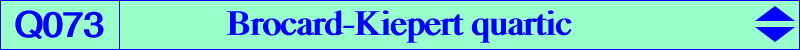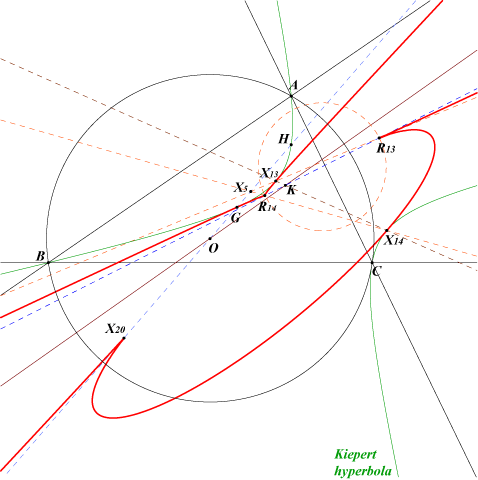too complicated to be written here. Click on the link to download a text file.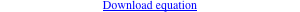X(2), X(13), X(14), X(20), X(3068), X(3069) R13 = X(3411) and R14 = X(3412) : see below X(39641), X(39642) are the imaginary foci of the Brocard ellipse i.e. common points of the Brocard axis and the Kiepert hyperbola X(42537) → X(42546)For any point M on the Brocard axis OK, the isogonal conjugate M* of M is a point on the Kiepert hyperbola. When M traverses OK, the line MM* envelopes the Brocard-Kiepert quartic Q073. See Table 38. Q073 has three cusps namely : – the de Longchamps point X(20) with tangent the Euler line, – R13 on the lines X(5)X(13), X(14)X(382), X(16)X(20), X(61)X(631), etc, with tangent the line X(5)X(13), – R14 on the lines X(5)X(14), X(13)X(382), X(15)X(20), X(62)X(631), etc, with tangent the line X(5)X(14). The first barycentric coordinates of these points are : a^2 S_A + 16 ∆^2 – 6√3 ∆ a^2 and a^2 S_A + 16 ∆^2 + 6√3 ∆ a^2 respectively, where ∆ is the area of ABC and S_A = (b^2+c^2-a^2)/2 as usual. R13 and R14 are now X(3411) and X(3412) in ETC (June 05, 2008). Note that the three cuspidal tangents are concurrent at X(5). Q073 is the locus of pivots of isogonal pivotal cubics that are tangent to the Brocard axis. This is in particular the case of K002 and K004. *** Q073 is a KHO-curve, see K1191 and CL075 for information. Its KHO-equation is : x^4 + 6 x^2 (22 y^2 - 14 y z + z^2) - 9 (2 y - z) (2 y + z)^3 = 0. See Q167, another KHO-quartic and the related Q183.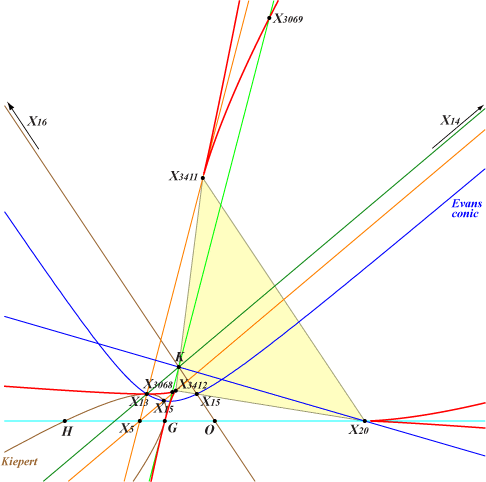In the KHO system of coordinates, most of the points involved have very simple coordinates. The three cusps are X(20) = (0,1,-2), X(3411) = (-9,2,5) and X(3412) = (9,2,5). The corresponding cuspidal tangents have equations x = 0 (Euler line), x - 3 (y - z) = 0 and x + 3 (y - z) = 0. The Brocard axis is y = 0 and the imaginary foci of the Brocard ellipse are (±i √3, 0, 1). They are double points on the curve. The line X(14)X(16) is x + y + z = 0, in a way the infinity line. The Kiepert hyperbola becomes a parabola with equation x^2 - 3 z (2y - z) = 0. The Evans conic is always a hyperbola with equation x^2 - 3 y^2 + 2 y z - z^2 = 0. The third polar of X(30) is the nodal cubic with node X(20) and KHO-equation : x^2 (29 y - 8 z) - 9 y (2 y + z)^2 = 0. It also passes through X(3), X(13), X(14), X(3411), X(3412).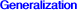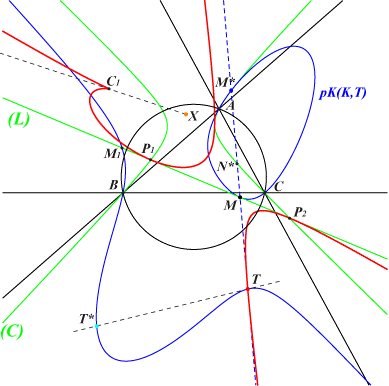Let (L) be a line and (C) its isogonal transform. A variable line passing through M on (L) and its isogonal conjugate M* on (C) meets (C) again at N*. Let T be the harmonic conjugate of N* with respect to M and M*. When M traverses (L), the line MM* envelopes a quartic (Q) and the point of tangency is T. (Q) is tricuspidal and the tangents at the three cusps (one is always real, see below) are concurrent at X which is the Ceva conjugate P/P* where P is the trilinear pole of (L). (Q) is bitangent to (L) at P1, P2 which are the (real or not) intersections of (L) and (C). (Q) is tangent to the 6 bisectors of ABC and to the sidelines of the anticevian triangle of the trilinear pole of (L). (Q) is the locus of the pivots of isogonal pivotal cubics which are tangent to (L). In other words, pK(K,T) is tangent at M to (L) and therefore tangent at M* to (C). *** A famous special case When (L) is the line at infinity, (C) is the circumcircle and (Q) is a deltoid, the anticomplement of the Steiner deltoid. (Q) is the envelope of the axes of parabolas inscribed in ABC. X is the circumcenter. In this case, T is the reflection of N* about M* and the points P1, P2 are the circular points at infinity. It follows that any pK(K,T) with T on this deltoid must be tangent at M to the line at infinity. This is the case of the cubic K695 for instance and also another analogous cubic mentioned in "Osculating circles on the Neuberg cubic".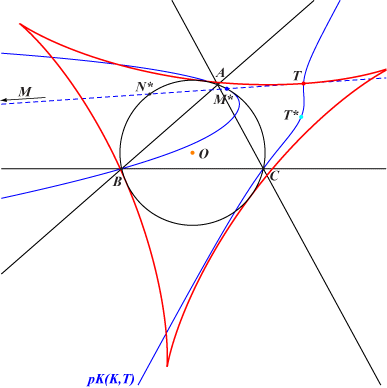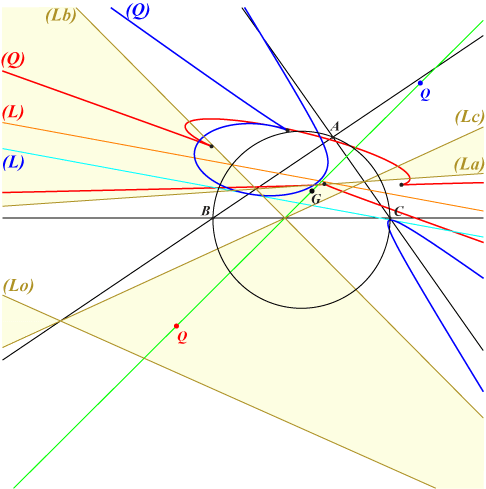Number of real cusps Let (L) be the line p x + q y + r z = 0 and let Q = p:q:r be the point which is the isotomic conjugate of the tripole P of (L). Consider the trilinear polar (Lo) of X(75) and its extraversions (La), (Lb), (Lc). These lines delimit several regions and when Q lies in one of them, the discriminant ∆ given by (ax+by+cz)(-ax+by+cz)(ax-by+cz)(ax+by-cz) keeps the same sign : – positive in the yellow regions, in particular those containing G and the vertices of the antimedial triangle, – negative in the white regions, in particular those containing A, B, C. When ∆>0, the quartic (Q) has three real cusps (red curve) and when ∆<0, it has only one real cusp (blue curve). When ∆=0, the quartic decomposes into a double line and a conic. This happens when (L) contains one in/excenter.